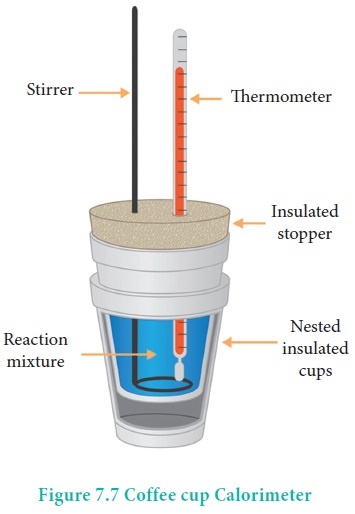Home | | Chemistry 11th std | Measurement of ╬öH using coffee cup calorimeter

# Measurement of ╬öH using coffee cup calorimeter

Heat change at constant pressure (at atmospheric pressure) can be measured using a coffee cup calorimeter.

╬öH Measurements

Heat change at constant pressure (at atmospheric pressure) can be measured using a coffee cup calorimeter. A schematic representation of a coffee cup calorimeter is given in Figure 7.7. Instead of bomb, a styrofoam cup is used in this calorimeter. It acts as good adiabatic wall and doesn't allow transfer of heat produced during the reaction to its surrounding. This entire heat energy is absorbed by the water inside the cup. This method can be used for the reactions where there is no appreciable change in volume. The change in the temperature of water is measured and used to calculate the amount of heat that has been absorbed or evolved in the reaction using the following expression.

q = mw Cw ╬öT

where mw is the molar mass of water and Cw is the molar heat capacity of water (4184 kJ K-1 mol-1)Problem 7. 4

Calculate the enthalpy of combustion of ethylene at 300 K at constant pressure, if its heat of combustion at constant volume (╬öU) is ŌłÆ1406 kJ .

The complete ethylene combustion reaction can be written as,

C2H4 (g) + 3O2(g) ŌåÆ 2CO2 (g)+2H2O(l)

╬öU = ŌłÆ1406 kJ

Ōłån = np(g) - nr(g)

Ōłån = 2 - 4 = -2

ŌłåH = ŌłåU + RTDng

ŌłåH  = -1406 + (8.314 ├Ś 10-3 ├Ś 300 ├Ś(-2)

ŌłåH = -1410.9 kJ

Study Material, Lecturing Notes, Assignment, Reference, Wiki description explanation, brief detail
11th Chemistry : UNIT 7 : Thermodynamics : Measurement of ╬öH using coffee cup calorimeter |